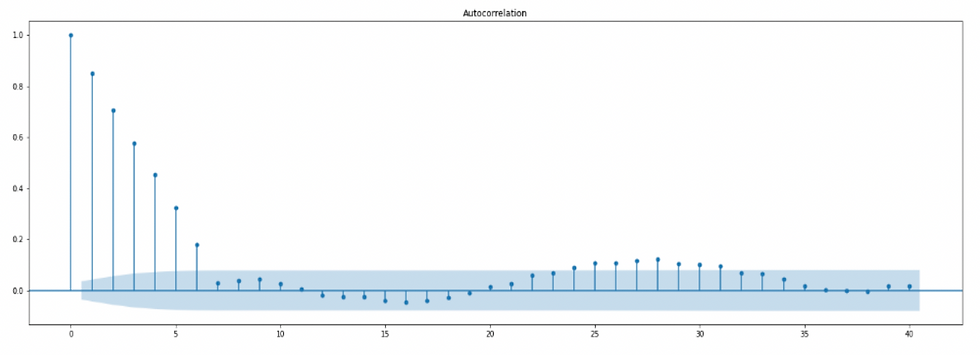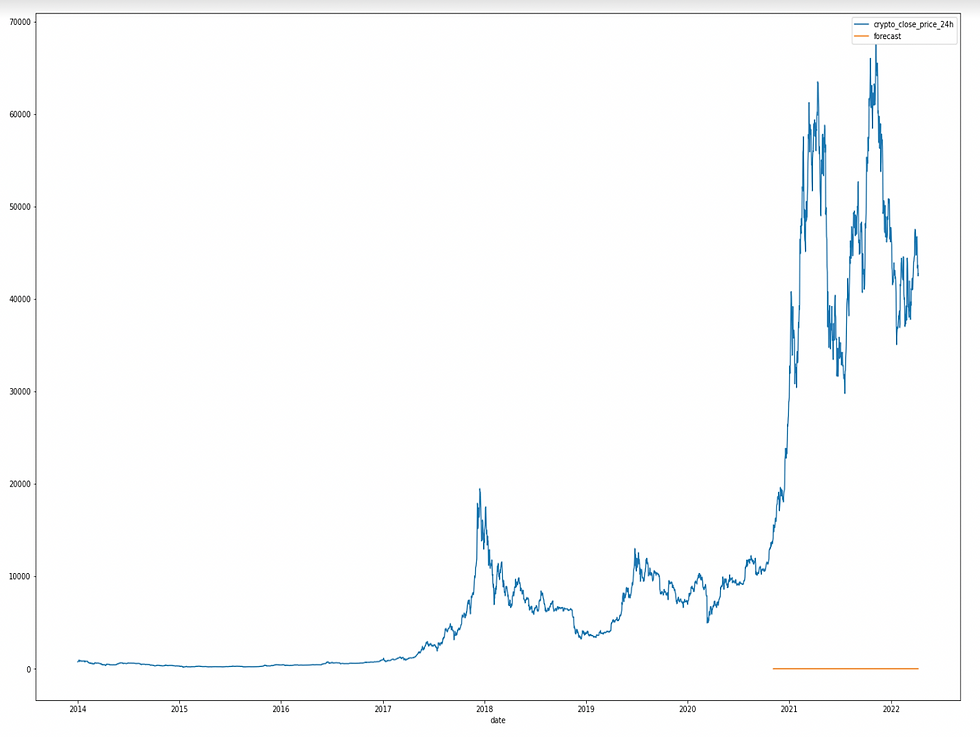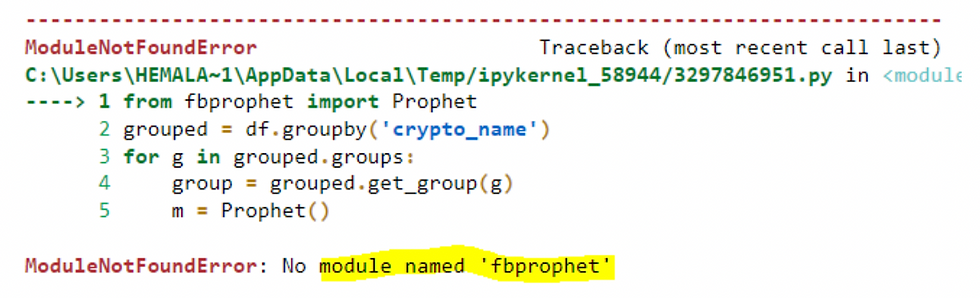top of page# Crypto Forecasting Using Python

Updated: Mar 9

Authors: Hemalatha, SS Prabhat & Anuja LohaniHere is the solution...!

##### Objective

To forecast future cryptocurrency prices, which will be helpful in estimating the returns.

##### Tools and packages used

Python, Snowflake python connector, matplotlib, ARIMA, SARIMA from statsmodel, Pandas, NumPy.

##### Introduction

A popular and widely used statistical method for time series forecasting is the ARIMA model. The two most popular methods for time series forecasting are exponential smoothing and ARIMA models offer complementary solutions to the issue.

Exponential smoothing methods are based on a description of the trend and seasonality in the data. Here The idea of stationarity and the technique of differencing time series method was applied.##### Stationarity

Time series with trends or seasonality are not stationary because stationary time series data has features that are independent of time. The trend and seasonality will affect the value of the time series at different times. However, for stationarity, it does not matter when you observe it; it should look much the same at any point in time. In general, a stationary time series won't often exhibit any long-term, predictable trends.

##### Model Description

ARIMA is an acronym that stands for Auto-Regressive Integrated Moving Average. It is a class of models that captures a suite of different standard temporal structures in time series data.

An ARIMA model is a class of statistical models for analyzing and forecasting time series data. It is really simplified in terms of using it, yet this model is really powerful. The parameters of the ARIMA (Auto-Regressive Integrated Moving Average) model are defined as follows:

p: The number of lag observations included in the model, also called the lag order.

d: The number of times that the raw observations are different, also called the degree of difference.

q: The size of the moving average window, also called the order of moving average.

A linear regression model is constructed, including the specified number and type of terms, and the data is prepared by a degree of differencing in order to make it stationary, i.e., to remove trend and seasonal structures that negatively affect the regression model.

##### Steps taken:

1. Visualize the Time Series Data.

2. Find out if the date is stationary.

3. Plot the Correlation and Autocorrelation Charts.

4. Construct the ARIMA Model or Seasonal ARIMA based on the data.##### To Generate the Dataset

1) Import the necessary modules and Install Snowflake Pythonconnector.

# import modules required for programming n plotting

import pandas as pd

import numpy as np

import matplotlib.pyplot as plt

2) ‘Get pass’ used to call encrypted password

Import getpass

pwd = getpass .getpass (“Enter password”)

#Create connection to Snowflake

import snowflake.connector

conn = snowflake.connector.connect (user='USER_NAME',

account='ACCOUNT LOCATION/ REGION',

#Create a variable called sql and specify a query that will store the result

then

sql= "select * from

STATIC_CRYPTO_DATA.COLLATED_STATIC_DATA.REPORTING_CR

YPTO_DATA"

3) Create the required Dataframe

df.info()

##### RESULT:4) Pick a group to do trial analyses first

Crypto group name-’Bitcoin’

#Use groupby() function to form groups based on more than one

category (crypto name then by dates)

gkk = df.groupby(['crypto_name'])

dataframe=gkk.get_group('Bitcoin')

5) Sort the data frame in the order of dates you want to analyze

dataframe_sort= dataframe.sort_values(by='date')

6) Select the required columns for the analyses-Subset data

dataframe_subset=dataframe_sort[["date","crypto_close_price_24h"]]

#dataframe_sort.describe()

#gkk.set_index('date',inplace=True)

dataframe_subset.columns=["date","crypto_close_price_24h"]

dataframe_subset.set_index('date',inplace=True)

from pylab import rcParams

rcParams['figure.figsize'] = 100, 50

dataframe_subset.plot()

8) X-axis-date, y-axis Closing Price9) Let’s check if the given dataset is stationary or not; for that, we use Ad

fuller Test from statsmodel lib in python

#STATISTICAL MODELLING

#Fuller test is to infer if the dataset is stationary or nonstationary

then we can perform Regression Model Forecasting

y=dataframe_subset['crypto_close_price_24h']

To identify the nature of the data, we will be using the null hypothesis.

H0: The null hypothesis: It is a statement about the population that either is believed to be true or is used to put forth an argument unless it can be shown to be incorrect beyond a reasonable doubt.

H1: The alternative hypothesis: It is a claim about the population that is contradictory to H0 and what we conclude when we reject H0.

#Ho: It is non-stationary

#H1: It is stationary

We will be considering the null hypothesis that data is not stationary and the alternate hypothesis that data is stationary.10) Let’s try to see the first difference and seasonal difference:

#any seasonal weekly difference

dataframe_subset['Price First Difference'] =

dataframe_subset['crypto_close_price_24h'] -

dataframe_subset['crypto_close_price_24h'].shift(1)

dataframe_subset['Seasonal weekly First

Difference']=dataframe_subset['crypto_close_price_24h']-

dataframe_subset['crypto_close_price_24h'].shift(7)

##### Output:11) Test the seasonally weekly difference values if it follows stationarityHere P-value is 4.73, which means we will be rejecting the null hypothesis. So, data is stationary.This means the data frame is ready for further analysis.

12) Next step is to create autocorrelation and partial autocorrelation plots Autocorrelation and partial autocorrelation are measures of association between current and past series values and indicate which past series values are most useful in predicting future values.

# Create Auto-Correlation

from pandas.plotting import autocorrelation_plot

autocorrelation_plot(dataframe_subset['crypto_close_price_24h'])

plt.show()13) For clarity's sake, generate Autocorrelation and Partial Autocorrelation plots.14) Import Warnings to avoid unnecessary abortion of the tasks executing

Import warnings

from statsmodels.tools.sm._exceptions import ConvergenceWarning

warnings.simplefilter(‘ignore’, ConvergenceWarning)

15) As Explained earlier now, we can apply ARIMA Model Train the data

# For non-seasonal data

#p=1, d=1, q=0 or 1

#p= order of the autoregressive part;

#d= degree of first differencing involved;

#q= order of the moving average part.

from statsmodels.tsa.arima_model import ARIMA

model=ARIMA(dataframe_subset['crypto_close_price_24h'],order=(1,1,1))

model_fit=model.fit()

model_fit.summary()It's very important to note down how many observations of each category are recorded so sample points for training can be used.

# TRAIN THE MODEL

dataframe_subset['forecast']=model_fit.predict(start=2500,end=3020,dynamic=True)

dataframe_subset[['crypto_close_price_24h','forecast']].plot(figsize=(24,16))16) Price and forecast for known observations are not aligned, so we need to apply Seasonal ARIMA, so it follows the trend. Here seven is for the weekly trend

import statsmodels.api as sm

model=sm.tsa.statespace.SARIMAX(dataframe_subset['crypto_close_price_

24h'],order=(1, 1, 1),seasonal_order=(1,1,1,7))

results=model.fit()

dataframe_subset['forecast']=results.predict(start=2500,end=3020,dynamic=

True)

dataframe_subset[['crypto_close_price_24h','forecast']].plot(figsize=(24,16))

dataframe_subset[['crypto_close_price_24h','forecast']]Remember the prediction should begin with respect to previous data points collected, the last observed value was 3021, so our prediction should begin from 3022 and end as per needed.

Example: Add 30 to the start point (if predicting for a month) and 14 to the start point(if predicting for two weeks time). Here (3022+30 ~ 3050 approx)

from pandas.tseries.offsets import DateOffset

future_dates=[dataframe_subset.index[-1]+ DateOffset(weeks=x)for x in

range(0,24)]

future_datest_df=pd.DataFrame(index=future_dates[1:],columns=dataframe_subs

et.columns)

future_datest_df.tail()

future_df=pd.concat([dataframe_subset,future_datest_df])

future_df['forecast'] = results.predict(start = 3021, end = 3050, dynamic= True)

future_df[['crypto_close_price_24h', 'forecast']].plot(figsize=(24, 16))

17) Predict the future values

These Forecasted trend lines are shown in orange color.18) List of Forecasted Price

##### FINAL OUTPUT:##### POST ANALYSES

Now this result can be either written to the snowflake or drawn out as a csv and used further for Analyses.

##### CHALLENGES

While exploring grouping instances, in spite of installing the packages, it gives no module-found error in the PC.

Example: fbprophet.Therefore the subset technique worked for our use case. Rest all the errors can be resolved if done meticulously.

##### CONCLUSION

Time Series forecasting is really useful when we have to make future decisions, or we have to do analysis; we can quickly do that using ARIMA. There are lots of other Models from which we can do the time series forecasting, but ARIMA is really easy to understand.

HAPPY CODING :)

##### REFERENCES

2) https://machinelearningmastery.com/time-series-data-stationary-python/

3) https://www.mygreatlearning.com/blog/time-series-analysis-and-forecasting/

4) https://stackoverflow.com/questions/50808322/prophet-fbprophet-package-in-python

5) https://towardsdatascience.com/multi-step-time-series-forecasting-with-arima-lightgbm-and-prophet-cc9e3f95dfb0